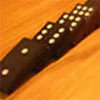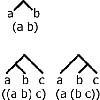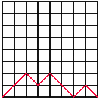# Resources tagged with: Mathematical induction

Filter by: Content type:
Age range:
Challenge level:

### There are 23 results

Broad Topics > Mathematical Thinking > Mathematical induction### OK! Now Prove It

##### Age 16 to 18 Challenge Level:

Make a conjecture about the sum of the squares of the odd positive integers. Can you prove it?### An Introduction to Mathematical Induction

##### Age 16 to 18

This article gives an introduction to mathematical induction, a powerful method of mathematical proof.### Binomial Coefficients

##### Age 14 to 18

An introduction to the binomial coefficient, and exploration of some of the formulae it satisfies.### Converging Product

##### Age 16 to 18 Challenge Level:

In the limit you get the sum of an infinite geometric series. What about an infinite product (1+x)(1+x^2)(1+x^4)... ?### Growing

##### Age 16 to 18 Challenge Level:

Which is larger: (a) 1.000001^{1000000} or 2? (b) 100^{300} or 300! (i.e.factorial 300)### Tens

##### Age 16 to 18 Challenge Level:

When is $7^n + 3^n$ a multiple of 10? Can you prove the result by two different methods?### Binary Squares

##### Age 16 to 18 Challenge Level:

If a number N is expressed in binary by using only 'ones,' what can you say about its square (in binary)?### Dirisibly Yours

##### Age 16 to 18 Challenge Level:

Find and explain a short and neat proof that 5^(2n+1) + 11^(2n+1) + 17^(2n+1) is divisible by 33 for every non negative integer n.### Elevens

##### Age 16 to 18 Challenge Level:

Add powers of 3 and powers of 7 and get multiples of 11.### Farey Fibonacci

##### Age 16 to 18 Short Challenge Level:

Investigate Farey sequences of ratios of Fibonacci numbers.### Gosh Cosh

##### Age 16 to 18 Challenge Level:

Explore the hyperbolic functions sinh and cosh using what you know about the exponential function.### Water Pistols

##### Age 16 to 18 Challenge Level:

With n people anywhere in a field each shoots a water pistol at the nearest person. In general who gets wet? What difference does it make if n is odd or even?### Farey Neighbours

##### Age 16 to 18 Challenge Level:

Farey sequences are lists of fractions in ascending order of magnitude. Can you prove that in every Farey sequence there is a special relationship between Farey neighbours?### Golden Powers

##### Age 16 to 18 Challenge Level:

You add 1 to the golden ratio to get its square. How do you find higher powers?### Obviously?

##### Age 14 to 18 Challenge Level:

Find the values of n for which 1^n + 8^n - 3^n - 6^n is divisible by 6.### One Basket or Group Photo

##### Age 7 to 18 Challenge Level:

Libby Jared helped to set up NRICH and this is one of her favourite problems. It's a problem suitable for a wide age range and best tackled practically.### Golden Fractions

##### Age 16 to 18 Challenge Level:

Find the link between a sequence of continued fractions and the ratio of succesive Fibonacci numbers.### Fibonacci Fashion

##### Age 16 to 18 Challenge Level:

What have Fibonacci numbers to do with solutions of the quadratic equation x^2 - x - 1 = 0 ?### Overarch 2

##### Age 16 to 18 Challenge Level:

Bricks are 20cm long and 10cm high. How high could an arch be built without mortar on a flat horizontal surface, to overhang by 1 metre? How big an overhang is it possible to make like this?### Particularly General

##### Age 16 to 18 Challenge Level:

By proving these particular identities, prove the existence of general cases.### Counting Binary Ops

##### Age 14 to 16 Challenge Level:

How many ways can the terms in an ordered list be combined by repeating a single binary operation. Show that for 4 terms there are 5 cases and find the number of cases for 5 terms and 6 terms.### Symmetric Tangles

##### Age 14 to 16

The tangles created by the twists and turns of the Conway rope trick are surprisingly symmetrical. Here's why!